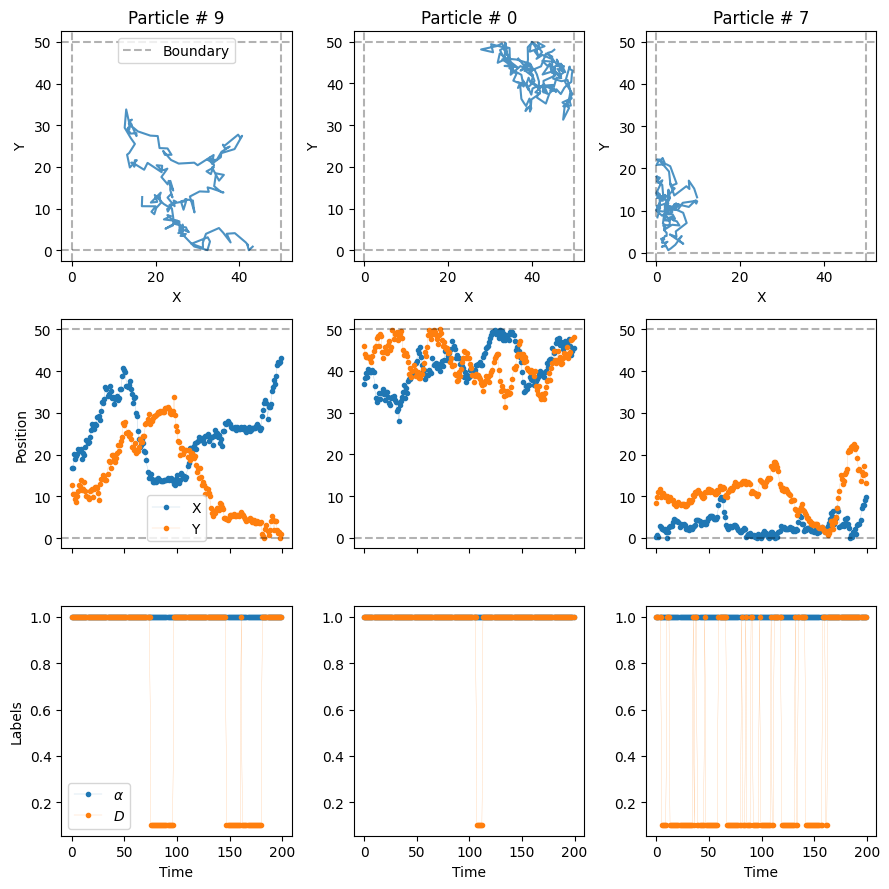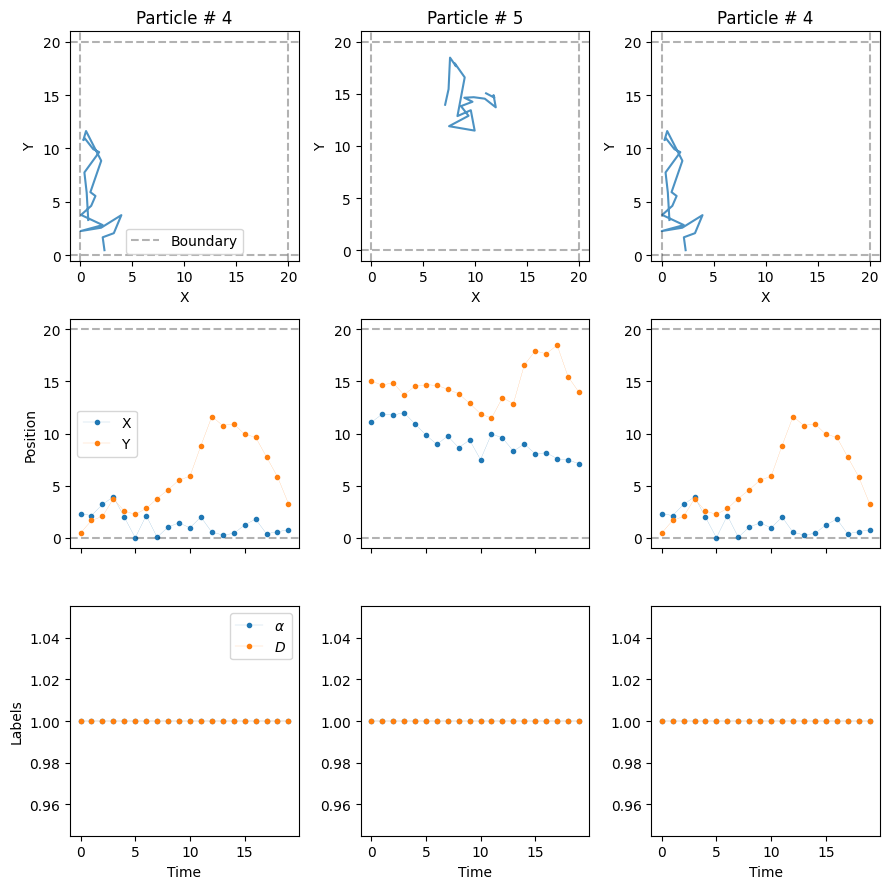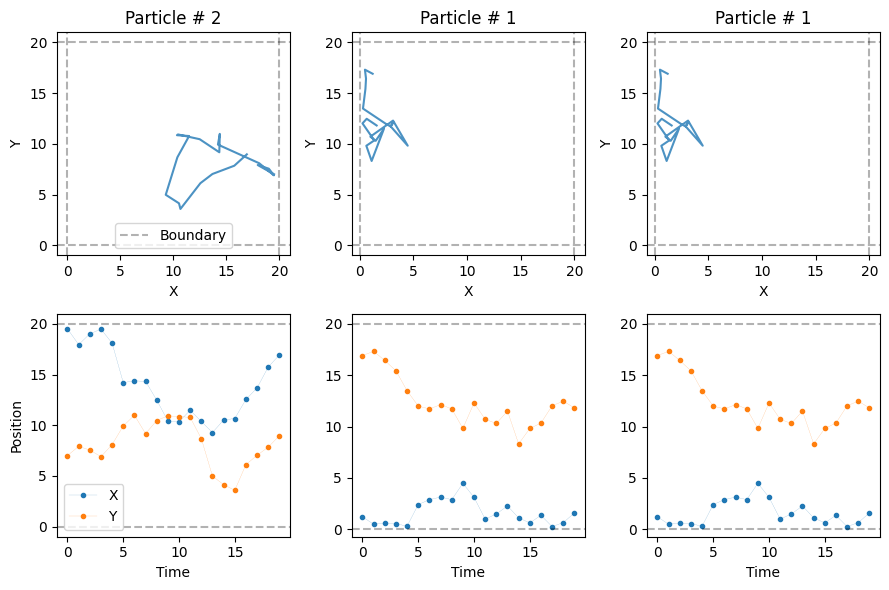source

### datasets_phenom

`````` datasets_phenom (models_class=<andi_datasets.models_phenom.models_phenom
object at 0x7f8fa1d92560>)``````

This class generates, saves and loads datasets of trajectories simulated from various phenomenological diffusion models (available at andi_datasets.models_phenom).

source

### create_dataset

`````` create_dataset (dics:list|dict|bool=False, T:None|int=None,
N_model:None|int=None, path:str='', save:bool=False,

Given a list of dictionaries, generates trajectories of the demanded properties. The only compulsory input for every dictionary is `model`, i.e. the model from which trajectories must be generated. The rest of inputs are optional. You can see the input parameters of the different models in `andi_datasets.models_phenom`, This function checks and handles the input dataset and the manages both the creation, loading and saving of trajectories.

Type Default Details
dics list | dict | bool False - if list or dictionary: the function generates trajectories with the properties stated in each dictionary.
- if bool: the function generates trajectories with default parameters set for the ANDI 2 challenge (phenom) for every available diffusion model.
T None | int None - if int: overrides the values of trajectory length in the dictionaries.
- if None: uses the trajectory length values in the dictionaries.
Caution: the minim T of all dictionaries will be considered!
N_model None | int None - if int: overrides the values of number of trajectories in the dictionaries.
- if None: uses the number of trajectories in the dictionaries
path str Path from where to save or load the dataset.
save bool False If True, saves the generated dataset (see self._save_trajectories).
Returns tuple - trajs (array TxNx2): particles’ position. N considers here the sum of all trajectories generated from the input dictionaries.
- labels (array TxNx2): particles’ labels (see ._multi_state for details on labels)

In the example below we create two dictionaries and generate a dataset with it. See the corresponding tutorial for more details.

``````L = 50
dict_model3 = {'model': 'dimerization',
'L': L,
'Pu': 0.1, 'Pb': 1}
dict_model5 = {'model': 'confinement',
'L': L,
'trans': 0.2}

dict_all = [dict_model3, dict_model5]

trajs, labels = datasets_phenom().create_dataset(N_model = 10, # number of trajectories per model
T = 200,
dics = dict_all
)
plot_trajs(trajs, L , N = 10,
num_to_plot = 3,
labels = labels,
plot_labels = True
)``````
``````False
False``````These auxiliary functions used in `create_trajectories` that allow for manipulate trajectories in various forms.

source

### _create_trajectories

`` _create_trajectories ()``

Given a list of dictionaries, generates trajectories of the demanded properties. First checks in the .csv of each demanded model if a dataset of similar properties exists. If it does, it loads it from the corresponding file.

``````L = 20
dict_1 = {'model': 'multi_state',
'L': L}
dict_2 = {'model': 'immobile_traps',
'L': L}
dict_all = [dict_1, dict_2]

DP = datasets_phenom()
trajs, labels = DP.create_dataset(N_model = 10, # number of trajectories per model
T = 20,
dics = dict_all
)
plot_trajs(trajs, L , N = 10,
num_to_plot = 3,
labels = labels,
plot_labels = True
)``````source

### _save_trajectories

`` _save_trajectories (trajs, labels, dic, df, dataset_idx, path)``

Given a set of trajectories and labels, saves two things:
- In the .csv corresponding to the demanded model, all the input parameters of the generated dataset. This allows to keed that of what was created before. - In a .npy file, the trajectories and labels generated.

``````trajs, labels = DP.create_dataset(N_model = 10, # number of trajectories per model
T = 20,
dics = dict_all,
save = True, path = 'datasets_folder/'
)
plot_trajs(trajs, L , N = 3)``````
``````C:\Users\Gorka\AppData\Local\Temp\ipykernel_11608\369869117.py:64: FutureWarning: The frame.append method is deprecated and will be removed from pandas in a future version. Use pandas.concat instead.
df = df.append(dic, ignore_index = True)
C:\Users\Gorka\AppData\Local\Temp\ipykernel_11608\369869117.py:64: FutureWarning: The frame.append method is deprecated and will be removed from pandas in a future version. Use pandas.concat instead.
df = df.append(dic, ignore_index = True)``````source

`` _load_trajectories (model_name, dataset_idx, path)``

Given the path for a dataset, loads the trajectories and labels

``````# You must run to cells above for this one to work. Check that this are the
# exact same trajectories.
trajs, labels = DP.create_dataset(N_model = 10, # number of trajectories per model
T = 20,
dics = dict_all,
load = True, path = 'datasets_folder/'
)
plot_trajs(trajs, L , N = 3 )``````# Managing parameters and dictionaries

``df = pd.read_csv('datasets_folder/immobile_traps.csv', index_col=0)``
``df``
N T L r Pu Pb Ds alphas Nt traps_pos deltaT model
0 10 20 10 1 0.1 0.01 [1, 0] [1, 0] 10 NaN 1 immobile_traps
``df.fillna('',inplace=True)``
``pd.notnull(df)``
N T L r Pu Pb Ds alphas Nt traps_pos deltaT model
0 True True True True True True True True True False True True
``df_conditions = df.where(pd.notnull(df), None)``
``df_conditions['traps_pos']``
``````0   NaN
Name: traps_pos, dtype: float64``````
``````dictm = {'model': 'immobile_traps',
'L': 10}

DP = datasets_phenom()
DP.N_model = 10
DP.T = 20
DP.save = False
DP.path = 'datasets_folder/'``````
``DP._inspect_dic(copy.deepcopy(dictm))``
``````(    N   T   L  r   Pu    Pb      Ds  alphas  Nt  traps_pos  deltaT  \
0  10  20  10  1  0.1  0.01  [1, 0]  [1, 0]  10        NaN       1

model
0  immobile_traps  ,
0)``````

source

### _inspect_dic

`` _inspect_dic (dic)``

Checks the information of the input dictionaries so that they fulfil the constraints of the program , completes missing information with default values and then decides about loading/saving depending on parameters.

Type Details
dic dict Dictionary with the information of the trajectories we want to generate
Returns tuple df: dataframe collecting the information of the dataset to load.
dataset_idx: location in the previous dataframe of the particular dataset we want to generate.

source

### _get_args

`` _get_args (model, return_defaults=False)``

Given the name of a diffusion model, return its inputs arguments.

Type Default Details
model str Name of the diffusion model (see self.available_models_name)
return_defaults bool False If True, the function will also return the default values of each input argument.
Returns tuple args (list): list of input arguments.
defaults (optional, list): list of default value for the input arguments.